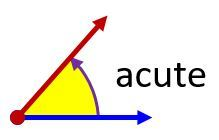# Angles

An angle is a figure formed by two rays that have the same endpoint.To name an angle, we use the symbol ∠ and three points names, listing the vertex in the middle. This is angle BAC or ∠BAC.

An angle is a fraction of a circle where the whole circle is 360°

The basic unit of measure for angles is the degree. One degree is 1/360 of a circle.

There are many different types of angles.

360° is a full turn  (complete rotation)180° is a half-turn (straight angle)90° is a quarter-turn  (right angle)The measure of a right angle is exactly 90°

The measure of an acute angle is greater than 0°  and less than 90°The measure of an obtuse angle is greater than 90°  and less than 180°The measure of a reflex angle is greater than 180°  and less than 360°A protractor is a device for measuring the angle between two rays.

Set the protractor so that one leg of the angle lies at zero, and the vertex of the angle at the center of the protractor. Read the angle value at the point where the other leg of the angle crosses the protractor.### Classifying Angles### AN21     Identify AnglesAngles_Hlp

Angle relationships

Congruent Angles have the same angle measure (one angle can be exactly superimposed on the other)

Complementary Angles are two angles whose values add up to 90 degrees.Supplementary Angles are two angles whose values add up to 180 degrees.Example. Two angles are supplementary. One angle is 20 larger than the other. Find the measures of both angles.Let’s say the small angle is X and the large angle is Y.

We know that supplementary angles add up to 180 degrees.  So, X+Y = 180°

The larger angle Y, is 20 degrees larger than the small one X.  This means Y = X + 20°

With this information, we can set up an equation.    X +(X+20°) =180°

Now, we can combine like terms and solve for X (the smaller angle).

2X+20° =180°        [subtract 20° from each side]

2X = 160°               [and divide both sides by 2]

X = 80°

Finally, add 20° to smaller angle X to find the larger angle Y

Y= X+20° = 80° + 20° = 100°

### Y = 100°### AN15        Missing Angle

Angles Vocabulary